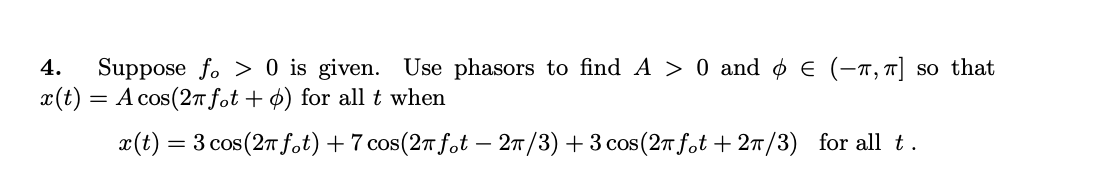Home / Answered Questions / Other / 4-suppose-fo-0-is-given-use-phasors-to-find-a-0-and-0-1-so-that-x-t-a-cos-27-fot-for-all-t-when-x-t--aw691

# (Solved): 4. Suppose Fo> 0 Is Given. Use Phasors To Find A > 0 And 0 â‚¬ (-1, So That X(t) = A Cos(27 Fot+Âº) ...4. Suppose fo> 0 is given. Use phasors to find A > 0 and 0 â‚¬ (-1, so that x(t) = A cos(27 fot+Âº) for all t when X(t) = 3 cos(27 f.t) + 7 cos(27 fot â€“ 27/3) + 3 cos(26 fot + 27/3) for all t.

We have an Answer from Expert